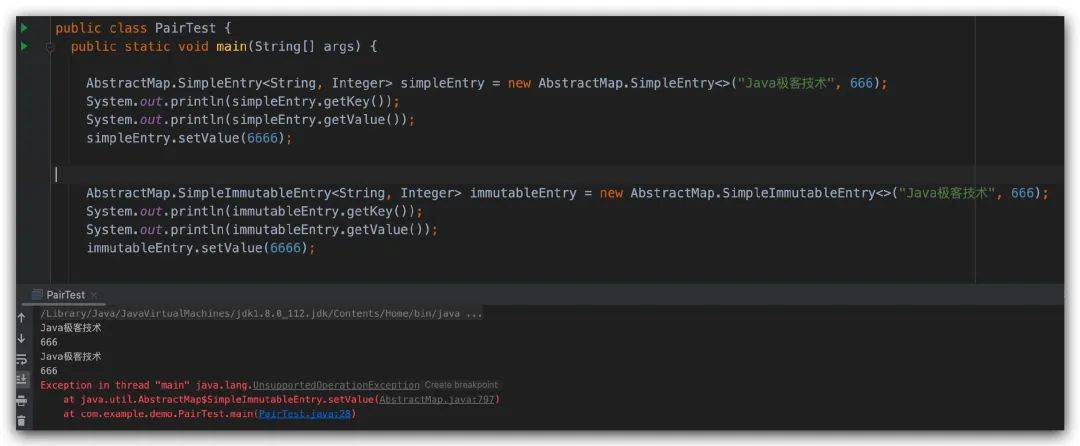# 我敢打赌你一定没用过 Java 中的这个类！

nill0705

0收藏

Python 示例：

``````#! /usr/bin/env python3
# -*- coding: utf-8 -*-

"""

"""

__author__ = 'ziyou'

def mul_result(a, b):
key = "key"
value = a + b
return key, value

if __name__ == '__main__':
key, mul = mul_result(3, 4)
print(key)
print(mul)``````

GO 示例：

``````package main

import "fmt"

func mul_result(a, b int) (key string, value int) {
key = "key"
value = a + b
return key, value
}

func main() {
key, value := mul_result(3, 4)
fmt.Println(key)
fmt.Println(value)
}``````

mul_result 函数接收两个入参，并且同时也返回两个结果，在调用的地方可以直接获取到两个返回值。

``````public static void main(String[] args) {
Map<String, Object> result = mul_result(3, 4);
System.out.println(result.get("key"));
System.out.println(result.get("value"));
}

public static Map<String, Object> mul_result(int a, int b) {
Map<String, Object> result  = new HashMap<>();
result.put("key", "key");
result.put("value", a + b);
return result;
}``````

``````public static void main(String[] args) {
Pair<String, Integer> pair = testPair(3, 4);
System.out.println(pair.getKey());
System.out.println(pair.getValue());
}

public static Pair<String, Integer> testPair(int a, int b) {
Pair<String, Integer> pair = new Pair<>("key", a + b);
return pair;
}``````

``````public static void main(String[] args) {

AbstractMap.SimpleEntry<String, Integer> simpleEntry = new AbstractMap.SimpleEntry<>("Java极客技术", 666);
System.out.println(simpleEntry.getKey());
System.out.println(simpleEntry.getValue());
simpleEntry.setValue(6666);

AbstractMap.SimpleImmutableEntry<String, Integer> immutableEntry = new AbstractMap.SimpleImmutableEntry<>("Java极客技术", 666);
System.out.println(immutableEntry.getKey());
System.out.println(immutableEntry.getValue());
immutableEntry.setValue(6666);

}``````Pair 类的使用场景主要就是在一些需要返回固定配对的地方，真正遇到的场景可能也不太多，所以大家在日常的工作中也并不常用，而且可替代的方案也有很多。

Javatuples 是一个第三方的 Java 元组库，这个库目前实现了最多十个元组，如果要实现常用的三元组或者四元组可以使用这个组件，不过更多元组的使用场景并不多，而且也不是很灵活，还是乖乖自定义实体吧。

``````<dependency>
<groupId>org.javatuples</groupId>
<artifactId>javatuples</artifactId>
<version>1.2</version>
</dependency>``````

``````Unit<A> (1 element)
Pair<A,B> (2 elements)
Triplet<A,B,C> (3 elements)
Quartet<A,B,C,D> (4 elements)
Quintet<A,B,C,D,E> (5 elements)
Sextet<A,B,C,D,E,F> (6 elements)
Septet<A,B,C,D,E,F,G> (7 elements)
Octet<A,B,C,D,E,F,G,H> (8 elements)帖子
视频
声望
粉丝
社区精华内容